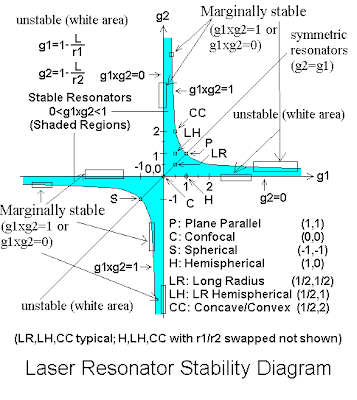Monday, November 22, 2010

Stability diagram of LASER resonator

L is resonator length.
r1 and r2 are radiuses of mirrors.
(∨ means 'or')

Stability condition is given by 0≤(1-L/r1)(1-L/r2)≤1

->Stable resonators fall in range: (1-L/r1)(1-L/r2)∈(0,1)

->Marginally stable are for: (1-L/r1)(1-L/r2)=0 ∨ (1-L/r1)(1-L/r2)=1

->Unstable ones are for (1-L/r1)(1-L/r2)<0 ∨ (1-L/r1)(1-L/r2)>11.